• c++实现最大（插入、删除、读取堆顶元素
2020-05-21 16:24:41

用数组来实现堆，数组序号为0的元素即为堆顶。
插入：将数据放到数组的最后，然后向上渗透。
删除堆顶：将数组最后一个元素替换堆顶，然后向下渗透。

头文件MaxHeap.h

#ifndef _MAX_HEAP_
#define _MAX_HEAP_

template <class T>
class MaxHeap
{
public:
MaxHeap(int mx = 10); //默认堆的最大值为10
virtual ~MaxHeap();

bool IsEmpty(); //判断堆是否为空
void Push(const T& e); //向堆中插入数据
void Pop();           // 删除堆顶
const T& Top() const;  //读取堆顶的值

private:
T* heapArray;
int maxSize; //堆的最大值
int currentSize; //堆的当前大小
void trickleUp(int index); //向上渗透
void trickleDown(int index); //向下渗透
};

template<class T>
MaxHeap<T>::MaxHeap(int mx = 10)
{
if (mx < 1) throw"max size must be >=1";
maxSize = mx;
currentSize = 0;
heapArray = new T[maxSize];
}

template<class T>
MaxHeap<T>::~MaxHeap()
{
delete[] heapArray;
}

template<class T>
bool MaxHeap<T>::IsEmpty()
{
return currentSize == 0;
}

template<class T>
void MaxHeap<T>::Push(const T& e)
{
if (currentSize == maxSize) throw"is full";
heapArray[currentSize] = e; //在数组尾部插入e
trickleUp(currentSize);  //向上渗透
currentSize++; //插入后堆当前大小 加1
}

template<class T>
void MaxHeap<T>::trickleUp(int index)
{
int parent = (index - 1) / 2; //当前节点的父亲节点
T bottom = heapArray[index];  //bottom 保存待插入节点
while (index > 0 && heapArray[parent] < bottom)
{
heapArray[index] = heapArray[parent];
index = parent;
parent = (parent - 1) / 2;
}
heapArray[index] = bottom;
}

template<class T>
const T& MaxHeap<T>::Top() const
{
return heapArray;
}

template<class T>
void MaxHeap<T>::Pop()
{
heapArray = heapArray[--currentSize];
trickleDown(0);

}

template<class T>

void MaxHeap<T>::trickleDown(int index)
{
int largeChild;
T top = heapArray[index];

while (index < currentSize / 2)
{
int leftChild = 2 * index + 1;
int rightChild = 2 * index + 2;
if (rightChild < currentSize && heapArray[leftChild] < heapArray[rightChild])
{
largeChild = rightChild;

}
else
{
largeChild = leftChild;
}
if (top >= heapArray[largeChild])
break;
heapArray[index] = heapArray[largeChild];
index = largeChild;
}
heapArray[index] = top;
}

#endif // !_MAX_HEAP_



测试代码：

#include<iostream>
#include"MaxHeap.h"

using namespace std;

int main()
{
MaxHeap<int> h(100);
h.Push(20);
h.Push(30);
h.Push(15);

cout << h.Top() << endl;
h.Pop();
cout << h.Top() << endl;
cin.get();
return 0;
}数据结构
更多相关内容
• class MaxHeap { int* h; int currentsize; int maxsize; public: MaxHeap(int *a,int n,int max); void BuildHeap(); void siftdown(int i); void siftup(int i); void delettop();... void show()
• 总是满足一下特性： 1.中某个结点的值总是不大于或不小于其父结点的值。 2.总是一棵完全二叉树。 一般用数组描述结构并用下标对其进行操作。 完全二叉树的数组形式就是将节点按层依次放入数组中。 比如下图...

只是一个鶸用来记录学习内容的文章罢了，如果能多少帮到你那真是太好了，发现错误欢迎指正。

堆总是满足一下特性：
1.堆中某个结点的值总是不大于或不小于其父结点的值。
2.堆总是一棵完全二叉树。
一般用数组描述堆结构并用下标对其进行操作。

完全二叉树的数组形式就是将节点按层依次放入数组中。
比如下图中的树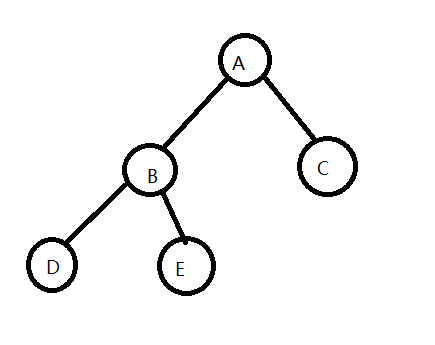用数组描述就是这样的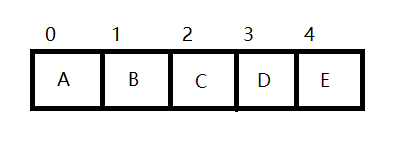由此不难发现：
若已知父节点下标x，则左孩子下标为x* 2+1，右孩子下标为x* 2+2。
若已知孩子下标为y，则其父节点下标为(y-1)/2。因为下标都是int类型所以右孩子按照此法算出来跟左孩子一样也是对的。

大顶堆就是父节点都大于孩子节点的堆，小顶堆就是父节点都小于孩子节点的堆。

# 插入元素

插入元素的过程中需要保证每次插入元素之后堆的结构都不被破坏。

方法是先将待插入元素添加至数组末尾（用的是STL的双端数组deque），然后从堆最末尾也就是完全二叉树的叶子节点向上探测看是否出现不满足堆特性的情况。

先初始化current和parent作为当前结点和当前结点的父节点的下标，比较父节点和待插入元素，若不符合堆特性（详情见注释）则用父节点的值覆盖当前节点，然后继续重复此动作直到找到待插入位置或当前结点没有父节点结束循环。这样父子节点就根据大小关系排好了。

void heap_insert(deque<int>* heap,int insert_number)
{
heap->push_back(insert_number);//先将元素插在堆末尾
int current = heap->size()-1,parent;
while(current > 0)
{//从下往上检验是否满足堆的特性
parent = (current-1)/2;
//以大顶堆为例，寻找新元素真正的插入位置时需要一直向上探测，比较待插入元素和当前节点的父节点大小如何
//如果待插入元素比当前结点的父节点还要大则父节点覆盖当前结点，然后当前结点指针继续向上，循环步骤
if(insert_number > heap->at(parent))
heap->at(current) = heap->at(parent);//当前结点的父节点覆盖当前结点
//如果待插入元素不比当前结点的父节点大，则当前结点即为待插入的位置，跳出循环
else
break;
current = parent;//当前结点指针变为父节点，准备继续向上探测
}
//跳出循环则意味着找到了待插入位置，直接将当前结点处的值修改为待插入元素即可
heap->at(current) = insert_number;
}


# 删除元素（堆顶）

删除堆顶元素需要首先记录堆顶元素，之后将堆末尾最后一个元素覆盖为堆顶元素，然后从堆顶开始向下探测，若不符合堆特性（详情见注释）则交换父节点和子节点然后继续重复此动作，由此来保证删除元素后堆的结构保持不变，最后返回之前记录的堆顶元素。

int heap_pop(deque<int>* heap)
{
int ret = heap->at(0);//记录堆顶元素
//最后一个元素覆盖第一个元素
heap->at(0) = heap->at(heap->size()-1);
heap->pop_back();

int current = 0,left_child,right_child;
bool IsLeft;
while(1)
{
IsLeft = true;//左孩子大于右孩子么？
left_child = current*2+1;
right_child = current*2+2;
if(left_child >= (int)heap->size()-1)
break;//越界跳出循环
if(heap->at(left_child) < heap->at(right_child))
IsLeft = false;//右孩子大
if(IsLeft && heap->at(current) < heap->at(left_child))
{//左孩子大且父节点小于左孩子，交换父节点和左孩子
swap(heap,current,left_child);
current = left_child;//更新当前结点
}
else if(!IsLeft && heap->at(current) < heap->at(right_child))
{//右孩子大且父节点小于右孩子，交换父节点和右孩子
swap(heap,current,right_child);
current = right_child;//更新当前结点
}
//因为每个节点都满足堆特性
//所以如果有一处的父节点大于所有的孩子则往下的所有的子节点都小于这个父节点因此可以不用往下看直接跳出循环
else
break;
}

return ret;
}


贴上所有测试代码

#include <bits/stdc++.h>
using namespace std;

void swap(deque<int>* heap,int a,int b)
{
int temp;
temp = heap->at(a);
heap->at(a) = heap->at(b);
heap->at(b) = temp;
}

void heap_insert(deque<int>* heap,int insert_number)
{
heap->push_back(insert_number);//先将元素插在堆末尾
int current = heap->size()-1,parent;
while(current > 0)
{//从下往上检验是否满足堆的特性
parent = (current-1)/2;
//以大顶堆为例，寻找新元素真正的插入位置时需要一直向上探测，比较待插入元素和当前节点的父节点大小如何
//如果待插入元素比当前结点的父节点还要大则父节点覆盖当前结点，然后当前结点指针继续向上，循环步骤
if(insert_number > heap->at(parent))
heap->at(current) = heap->at(parent);//当前结点的父节点覆盖当前结点
//如果待插入元素不比当前结点的父节点大，则当前结点即为待插入的位置
else
break;
current = parent;//当前结点指针变为父节点，准备继续向上探测
}
//跳出循环则意味着找到了待插入位置，直接将当前结点处的值修改为待插入元素即可
heap->at(current) = insert_number;
}

int heap_pop(deque<int>* heap)
{
int ret = heap->at(0);//记录堆顶元素
//最后一个元素覆盖第一个元素
heap->at(0) = heap->at(heap->size()-1);
heap->pop_back();

int current = 0,left_child,right_child;
bool IsLeft;
while(1)
{
IsLeft = true;//左孩子大于右孩子么？
left_child = current*2+1;
right_child = current*2+2;
if(left_child >= (int)heap->size()-1)
break;//越界跳出循环
if(heap->at(left_child) < heap->at(right_child))
IsLeft = false;//右孩子大
if(IsLeft && heap->at(current) < heap->at(left_child))
{//左孩子大且父节点小于左孩子，交换父节点和左孩子
swap(heap,current,left_child);
current = left_child;//更新当前结点
}
else if(!IsLeft && heap->at(current) < heap->at(right_child))
{//右孩子大且父节点小于右孩子，交换父节点和右孩子
swap(heap,current,right_child);
current = right_child;//更新当前结点
}
//因为每个节点都满足堆特性
//所以如果有一处的父节点大于所有的孩子则往下的所有的子节点都小于这个父节点因此可以不用往下看直接跳出循环
else
break;
}

return ret;
}

int peek(deque<int> heap)
{
if(heap.size() == 0)
{
cout<<"空堆"<<endl;
return -1;
}
return heap;
}

void show_heap(deque<int> heap)
{
cout<<"堆内部大致情况如下\n";
int line_sum = 1,x = 1,line = 1;
for(int i = 1;i<(int)heap.size();i=i*2+1)
line_sum++;
for(int i = 0;i < line_sum;i++)
cout<<"  ";
for(int i = 0;i < (int)heap.size();i++)
{
cout<<heap[i]<<" ";
if(i+1 == x)
{
cout<<endl;
for(int j = 0;j < line_sum-line;j++) cout<<"  ";
x = x*2+1;
}
line++;
}
cout<<endl;
}

int main()
{
deque<int> heap;

printf("请输入待放入堆中的元素，以-1结尾\n");
int in;
while(1)
{
cin>>in;
if(in == -1) break;
heap_insert(&heap,in);
}
show_heap(heap);
while(1)
{
if(heap.size() == 0) break;
cout<<"  "<<heap_pop(&heap)<<" "<<endl;
show_heap(heap);
}
return 0;
}


展开全文数据结构 二叉树
• ## C++大顶堆和小顶堆

千次阅读 2020-11-24 09:04:59
C++大顶堆和小顶堆原理大顶堆顶堆大顶堆和小顶堆对比图大顶堆和小顶堆的实现代码 原理   数据结构是一种数组对象，它可以被视为一颗完全二叉树结构（或者也有可能是满二叉树） 大顶堆   根结点（亦称为堆顶...

# 原理

堆数据结构是一种数组对象，它可以被视为一颗完全二叉树结构（或者也有可能是满二叉树）

## 大顶堆

根结点（亦称为堆顶）的关键字是堆里所有结点关键字中最大者，称为大顶堆。大根堆要求根节点的关键字既大于或等于左子树的关键字值，又大于或等于右子树的关键字值。

## 小顶堆

根结点（亦称为堆顶）的关键字是堆里所有结点关键字中最小者，称为小顶堆。小根堆要求根节点的关键字既小于或等于左子树的关键字值，又小于或等于右子树的关键字值。

## 大顶堆和小顶堆对比图## 大顶堆和小顶堆的实现代码

heap.h

#pragma once
#include<iostream>
#include<assert.h>
#include<vector>
using namespace std;

template<class T>
struct Less
{
bool operator()(const T& left, const T& right) const
{
return left < right;
}
};

template<class T>
struct Greater
{
bool operator()(const T& left, const T& right) const
{
return left > right;
}
};

template<class T, class Compare = Less<T>>
class Heap
{
public:
Heap()//无参的构造函数（系统不会给无参构造函数），开始堆是空的不需要做什么事
{}
Heap(T* a, size_t n)
{
_a.reserve(n);//开空间
for (size_t i = 0; i < n; ++i)
{
_a.push_back(a[i]);

}
//建堆,找最后一个非叶子节点
for (int i = (_a.size() - 2) / 2; i >= 0; --i)//不用size_t，因为i在这可能等于0，用size_t会死循环
{
}
}
//向下调整
{
Compare com;
int parent = root;
size_t child = parent * 2 + 1;//默认为左孩子
while (child < _a.size())
{
//选出小孩子
//if (child+1 > _a.size() && _a[child + 1]< _a[child])
if (child + 1 < _a.size() && com(_a[child + 1], _a[child]))
{
++child;
}

//if (_a[child] < _a[parent])
if (com(_a[child], _a[parent]))
{
swap(_a[child], _a[parent]);//交换值
parent = child;
child = parent * 2 + 1;
}
else
{
break;
}
}
}
//向上调整
{
Compare com;
int parent = (child - 1) / 2;
while (parent >= 0)
{
//if (_a[child] < _a[parent])
if (com(_a[child], _a[parent]))
{
swap(_a[parent], _a[child]);
child = parent;
parent = (child - 1) / 2;
}
else
{
break;
}
}

}
//最后插入
void Push(const T&x)
{
_a.push_back(x);
}
//删除最大数
void Pop()
{
assert(!_a.empty());
swap(_a, _a[_a.size() - 1]);
_a.pop_back();

}
//取顶元素
T& Top()
{
assert(!_a.empty());
return _a;
}
size_t Size()
{
return _a.size();
}

bool Empty()
{
return _a.empty();
}

private:
vector<T> _a;

};


main.cpp

#include <iostream>
#include "heap.h"
using namespace std;

int main()
{
int a[] = { 10,11,13,12,16,18,15,17,14,19 };
// Heap<int,Greater<int>> hp1(a,sizeof(a)/sizeof(a)); 最大堆
// Heap<int,Less<int>> hp1(a,sizeof(a)/sizeof(a)); 最小堆
Heap<int> hp1(a, sizeof(a) / sizeof(a)); // 缺省，最小堆
hp1.Push(15);
system("pause");
return 0;
}


# vector和push_heap、pop_heap实现堆

## 建堆

vector<int> nums = {9, 6, 2, 4, 7, 0, 1, 8, 3, 5};


如何使用nums构建最大堆

make_heap(nums.begin(), nums.end());
//或
make_heap(nums.begin(), nums.end(), less<int>());


如何使用nums构建最小堆

make_heap(nums.begin(), nums.end(), greater<int>());


## 调整堆

当使用上述的make_heap()建完堆后，如果vector使用push_back()插入数据或pop_back()删除数据后，会破坏最大堆/最小堆的性质，所以需要调整堆，常用push_heap()和pop_heap()两个方法。
1、push_heap()用法是，vector先push_back()，后push_heap()

nums.push_back(10);
push_heap(nums.begin(), nums.end(), less<int>());


2、pop_heap()用法是，先pop_heap()，vector后pop_back()

pop_heap(nums.begin(), nums.end(), less<int>());
nums.pop_back();


为什么pop_heap()的用法要反过来呢？
要从我们的目的来考虑，使用pop_heap()的绝大部分目的是要把堆顶元素pop出堆中，因为它最大或最小。如果先用vector的pop_back()，它删除的不是堆顶元素（nums），而是vector的最后一个元素。可见这不是我们想要的结果：对于最大堆，最后一个元素既不是最大，也不一定是最小；对于最小堆，最后一个元素既不是最小，也不一定是最大。pop出来没有意义。
观察pop_heap()对堆做了什么？
pop_heap()把堆顶元素放到了最后一位，然后对它前面的数字重建了堆。这样一来只要再使用pop_back()把最后一位元素删除，就得到了新的堆。

# priority_queue实现堆

priority_queue
对于这个模板类priority_queue，它是STL所提供的一个非常有效的容器。
作为队列的一个延伸，优先队列包含在头文件 中。

## 简述

优先队列时一种比较重要的数据结构，它是有二项队列编写而成的，可以以O(log n) 的效率查找一个队列中的最大值或者最小值，其中是最大值还是最小值是根据创建的优先队列的性质来决定的。

## 模板参数

优先队列有三个参数，其声明形式为：

priority_queue< type, container, function >


这三个参数，后面两个可以省略，第一个不可以。其中：
**type：**数据类型；
**container：**实现优先队列的底层容器；
**function：**元素之间的比较方式；
对于container，要求必须是数组形式实现的容器，例如vector、deque，而不能使list。
在STL中，默认情况下（不加后面两个参数）是以vector为容器，以 operator< 为比较方式，所以在只使用第一个参数时，优先队列默认是一个最大堆，每次输出的堆顶元素是此时堆中的最大元素。

## 成员函数

假设type类型为int，则：

1. bool empty() const 返回值为true，说明队列为空；
2. int size() const 返回优先队列中元素的数量；
3. void pop() 删除队列顶部的元素，也即根节点；
4. int top() 返回队列中的顶部元素，但不删除该元素；
5. void push(int arg) 将元素arg插入到队列之中。

## 大顶堆和小顶堆

#include <functional>

//构造一个空的优先队列（此优先队列默认为大顶堆）
priority_queue<int> big_heap;
//另一种构建大顶堆的方法
priority_queue<int,vector<int>,less<int> > big_heap2;

//构造一个空的优先队列,此优先队列是一个小顶堆
priority_queue<int,vector<int>,greater<int> > small_heap;

展开全文• 大小顶堆的实现什么是大顶堆和小顶堆大小顶堆的底层实现代码实现小顶堆定义小顶堆类构造函数插入扩大数组容量删除析构函数代码实现大顶堆测试 什么是大顶堆和小顶堆 是一种完全二叉树。完全二叉树的定义：所有...

# 什么是大顶堆和小顶堆

堆是一种完全二叉树。完全二叉树的定义：所有节点从上往下，从左往右的依次排列，不能有空位置，是为完全二叉树。下面是完全二叉树和不完全二叉树的示意图：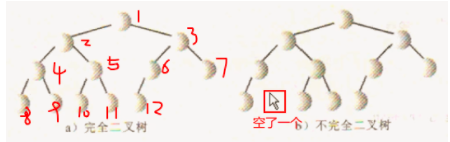大顶堆定义：父节点都大于左右子节点。
小顶堆定于：父节点都小于左右子节点。
二者示意图如下：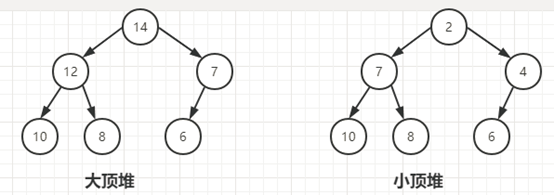# 大小顶堆的底层实现

一般来说，定义二叉树都是定义一个节点类，节点类中包含本节点的数据元素和两个节点指针，分别指向左右子节点，通过这两个节点指针将整个树节点连起来。如下：

template <typename Type>
class BstNode
{
public:
BstNode():leftChild(nullptr), rightChild(nullptr){};
BstNode(Type _content) :leftChild(nullptr), rightChild(nullptr)
{
content = _content;
};
private:
Type content;
BstNode<Type> *leftChild;
BstNode<Type> *rightChild;
};


实际上，堆一般用数组表示，如下图。究其原因，我认为的是通过节点类的方式占用的内存空间多，多加了两个指针。并且当我们做插入操作向上渗透时，需要找到节点的父节点(文章后面会详细描述)，这样实际的节点应该再加一个标识父节点的指针。这样实际是多了3个指针。（Ps：当然，我的水平也有限，只能看到这一层，如果不对的话或者还有其他原因，欢迎批评指正。）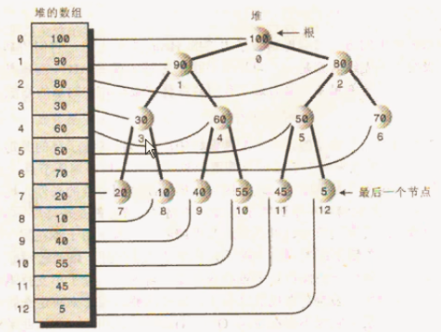# 代码实现小顶堆

## 定义小顶堆类

template<typename T>
class MinHeap
{
public:
MinHeap(int _maxsize = 10);
~MinHeap();

void Push(T _data);//插入数据
void Pop();//删除堆顶数据
T& Top();//获取堆顶数据

void ShowHeap();//打印堆
private:
T *heap;
int MaxSize;//堆数组的最大大小
int currentSize;//堆数组当前大小
};


## 构造函数

template<typename T>
MinHeap<T>::MinHeap(int _maxsize)
:MaxSize(_maxsize)
{
try{
if (MaxSize < 1)
throw "Error Size,< 1";
heap = new T[MaxSize];
currentSize = 0;
}
catch (const char* cp){

//1.提示用户错误信息，提示用户重新输入
int size = 0;
while (size < 1){
std::cout << cp << std::endl;
std::cout << "please Enter size again" << std::endl;
std::cin >> size;
}

//2.初始化MinHeap类的数据成员
MaxSize = size;
heap = new T[MaxSize];
currentSize = 0;
}
}


解释：这里我做了异常处理。当堆数组的大小MaxSize<1时，抛出异常，并提示输入大小，一直到输入的大小>=1。
关于C++的异常处理，参考：详解C++异常处理使用方法

## 插入

插入方法：

1. 首先将需插入的数据放在堆的最后一个位置
2. 然后依次和父节点比较，比父节点小就和父节点交换，再向上比较；比父节点大就停止比较。

比如我按20->30->15->10->9->8->12依次插入，插入示意图如下：代码如下：

template<typename T>
void MinHeap<T>::Push(T _data)
{
if (currentSize == MaxSize){
//堆满了，扩容
}
//将数据放入最后一个位置
//注意：currentSize和数组索引值差1：
//比如currentSize = 3，对应的数组索引为2
//所以，当currentSize=3时，即堆数组有3个数据时，新插入的数据应在数组索引3上
heap[currentSize] = _data;

int sonIndex = currentSize;//最后一个位置的数组索引
int fatherIndex = (currentSize - 1)/ 2;//最后一个位置的父节点的数组索引

while (sonIndex > 0){
if (heap[sonIndex] < heap[fatherIndex]){
std::swap(heap[sonIndex], heap[fatherIndex]);//交换父子节点元素

//继续向上
sonIndex = fatherIndex;
fatherIndex = (fatherIndex - 1) / 2;
}
else break;
}

currentSize++;//堆数组当前大小加1
}


这里简单解释一下：

1. currentSize表示的是当前堆数组有多少个元素。如下图，比如currentSize = 3，表明当前有3个数据。再push一个数据时，在数组的存储的位置刚好是索引3。
2. 如下图，节点7和节点4的父节点都是节点2。节点7的索引为1，节点4索引为2，节点2的索引为0，所以计算7 4 节点的父节点公式为==（索引-1）/2==，其他节点也一样。这里你不从数组索引角度，从树的层序角度也是一样，只不过公式要变为：层序号/2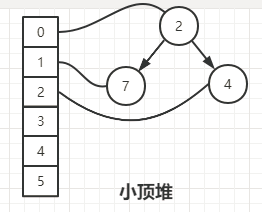### 扩大堆数组容量

在上述代码中，当currentSize == MaxSize时，表示堆数组已经满了，此时我们需要扩大数组的大小，这和循环队列和顺序栈是一样的原理。
循环队列：带你一步步用C++实现循环队列
顺序栈：用C++实现顺序栈(以数组为底层数据结构，可自动扩容)
代码如下：

template<typename T>
void MinHeap<T>::ChangeSize()
{
int size = MaxSize * 2;//堆数组容量扩大1倍

T* tmp = new T[size];//1.申请一块原来2倍大小的空间。
std::copy(heap, heap + MaxSize, tmp);//2.将栈中的数据赋值到新的内存空间
delete[] heap;//3.删除老的空间

heap = tmp;//4.heap指向新地址
MaxSize = size;//5.改变MaxSize
}


注意：由于C++编译器是不检查数组越界的，所以，即使程序员不自己扩容，使用越界的索引程序也不会报错，只是它会继续向下占用一块内存。程序员必须注意数组越界的问题。

std::copy()函数介绍：
使用std::copy时会报错，解决方法是：在预处理器加入(项目属性—-C/C++—-预处理—-预处理器定义)：_SCL_SECURE_NO_WARNINGS。

## 删除

删除方法：

1. 首先把堆顶元素删除
2. 接着把堆的最后一个数据放在堆顶
3. 最后把堆顶数据向下渗透，不断的和两个子节点比较，若父节点不比两个子节点的任意一个小，取两个子节点中小的和父节点交换，一直这样下去，直到父节点比左右子节点都小。

删除示意图如下：代码如下：

template<typename T>
void MinHeap<T>::Pop()
{
if (currentSize == 0){
std::cout << "Error!The heap is empty，Invalid operation." << std::endl;
return;
}

heap = heap[--currentSize];//删除堆顶，并将最后一个数据放在堆顶

int fatherIndex = 0;//父节点索引为堆顶
int leftSonIndex = fatherIndex * 2 + 1;//获得左子节点索引
int RightSonIndex = leftSonIndex + 1;//获得右子节点索引

//确认循环条件
while (leftSonIndex < currentSize ){
/**1.找左右子节点中最小值的数组索引***/
int minIndex;
//如果RightSonIndex < currentSize,表明fatherIndex有右子节点
if (RightSonIndex < currentSize){
minIndex = heap[leftSonIndex] > heap[RightSonIndex] ?  RightSonIndex: leftSonIndex;
}
else{
minIndex = leftSonIndex;
}

/**2.父节点大就交换，否则退出循环***/
if (heap[fatherIndex] > heap[minIndex])
std::swap(heap[fatherIndex], heap[minIndex]);
else break;

fatherIndex = minIndex;
leftSonIndex = fatherIndex * 2 + 1;
RightSonIndex = leftSonIndex + 1;
}
}


对于while循环条件说明：
使用左子节点leftSonIndex和currentSize比较，不要使用右子节点RightSonIndex，因为右子节点RightSonIndex可能没有。当然，你也可以使用父节点fatherIndex和currentSize比较：fatherIndex<currentSize/2。
验证循环的真确性，只需考虑以下三种情况，大家可自行验证。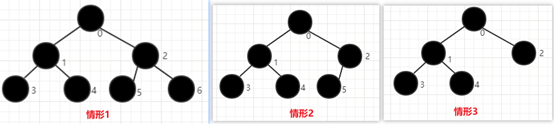## 析构函数

析构只需将申请的内存释放即可。

template<typename T>
MinHeap<T>::~MinHeap()
{
delete []heap;
}


# 代码实现大顶堆

原理和小顶堆一样，直接给出代码

#ifndef _MAX_HEAP_H
#define _MAX_HEAP_H

template<typename T>
class MaxHeap
{
public:
MaxHeap(int _maxsize = 10);
~MaxHeap();

void Push(T _data);//插入数据
void Pop();//删除堆顶数据
T& Top();//获取堆顶数据

void ShowHeap();
private:
T *heap;
int MaxSize;//堆数组的最大大小
int currentSize;//堆数组当前大小

void ChangeSize();//扩大堆数组的大小
};

template<typename T>
MaxHeap<T>::MaxHeap(int _maxsize)
:MaxSize(_maxsize)
{
try{
if (MaxSize < 1)
throw "Error Size,< 1";
heap = new T[MaxSize];
currentSize = 0;
}
catch (const char* cp){

//1.提示用户错误信息，提示用户重新输入
int size = 0;
while (size < 1){
std::cout << cp << std::endl;
std::cout << "please Enter size again" << std::endl;
std::cin >> size;
}

//2.初始化MaxHeap类的数据成员
MaxSize = size;
heap = new T[MaxSize];
currentSize = 0;
}
}
template<typename T>
MaxHeap<T>::~MaxHeap()
{
delete[]heap;
}
template<typename T>
void MaxHeap<T>::Push(T _data)
{
if (currentSize == MaxSize){
//堆满了，扩容
ChangeSize();
}
//将数据放入最后一个位置
//注意：currentSize和数组索引值差1：
//比如currentSize = 3，对应的数组索引为2
//所以，当currentSize=3时，即堆数组有3个数据时，新插入的数据应在数组索引3上
heap[currentSize] = _data;

int sonIndex = currentSize;//最后一个位置的数组索引
int fatherIndex = (currentSize - 1) / 2;//最后一个位置的父节点的数组索引

while (sonIndex > 0){
if (heap[sonIndex] > heap[fatherIndex]){
std::swap(heap[sonIndex], heap[fatherIndex]);

//继续向上
sonIndex = fatherIndex;
fatherIndex = (fatherIndex - 1) / 2;
}
else break;
}

currentSize++;
}
template<typename T>
void MaxHeap<T>::Pop()
{
if (currentSize == 0){
std::cout << "Error!The heap is empty，Invalid operation." << std::endl;
return;
}

heap = heap[--currentSize];//删除堆顶，并将最后一个数据放在堆顶

int fatherIndex = 0;//父节点索引为堆顶
int leftSonIndex = fatherIndex * 2 + 1;//获得左子节点索引
int RightSonIndex = leftSonIndex + 1;//获得右子节点索引

//确认循环条件
while (leftSonIndex < currentSize){
/**1.找左右子节点中最小值的数组索引***/
int maxIndex;
//如果RightSonIndex < currentSize,表明fatherIndex有右子节点
if (RightSonIndex < currentSize){
maxIndex = heap[leftSonIndex] < heap[RightSonIndex] ? RightSonIndex : leftSonIndex;
}
else{
maxIndex = leftSonIndex;
}

/**2.父节点小就交换，否则退出循环***/
if (heap[fatherIndex] < heap[maxIndex])
std::swap(heap[fatherIndex], heap[maxIndex]);
else break;

fatherIndex = maxIndex;
leftSonIndex = fatherIndex * 2 + 1;
RightSonIndex = leftSonIndex + 1;
}
}
template<typename T>
T& MaxHeap<T>::Top()
{
if (currentSize == 0)
throw "Error.The heap is empty,there is not data.";
return heap;
}

template<typename T>
void MaxHeap<T>::ChangeSize()
{
int size = MaxSize * 2;//堆数组容量扩大1倍

T* tmp = new T[size];//1.申请一块原来2倍大小的空间。
std::copy(heap, heap + MaxSize, tmp);//2.将栈中的数据赋值到新的内存空间
delete[] heap;//3.删除老的空间

heap = tmp;//4.heap指向新地址
MaxSize = size;//5.改变MaxSize
}

template<typename T>
void MaxHeap<T>::ShowHeap()
{
int size = 1;
for (int i = 0; i < currentSize;){
std::cout << "[ ";
for (int j = 0; j < size && i < currentSize; j++){
std::cout << heap[i++] << " ";
}
std::cout << "]" << std::endl;
size *= 2;
}
}

#endif


# 测试

下面只测试小顶堆，大顶堆可自行测试。
MinHeap.h

#ifndef _MIN_HEAP_H
#define _MIN_HEAP_H

template<typename T>
class MinHeap
{
public:
MinHeap(int _maxsize = 10);
~MinHeap();

void Push(T _data);//插入数据
void Pop();//删除堆顶数据
T& Top();//获取堆顶数据

void ShowHeap();
private:
T *heap;
int MaxSize;//堆数组的最大大小
int currentSize;//堆数组当前大小

void ChangeSize();//扩大堆数组的大小
};

template<typename T>
MinHeap<T>::MinHeap(int _maxsize)
:MaxSize(_maxsize)
{
try{
if (MaxSize < 1)
throw "Error Size,< 1";
heap = new T[MaxSize];
currentSize = 0;
}
catch (const char* cp){

//1.提示用户错误信息，提示用户重新输入
int size = 0;
while (size < 1){
std::cout << cp << std::endl;
std::cout << "please Enter size again" << std::endl;
std::cin >> size;
}

//2.初始化MinHeap类的数据成员
MaxSize = size;
heap = new T[MaxSize];
currentSize = 0;
}
}
template<typename T>
MinHeap<T>::~MinHeap()
{
delete []heap;
}
template<typename T>
void MinHeap<T>::Push(T _data)
{
if (currentSize == MaxSize){
//堆满了，扩容
ChangeSize();
}
//将数据放入最后一个位置
//注意：currentSize和数组索引值差1：
//比如currentSize = 3，对应的数组索引为2
//所以，当currentSize=3时，即堆数组有3个数据时，新插入的数据应在数组索引3上
heap[currentSize] = _data;

int sonIndex = currentSize;//最后一个位置的数组索引
int fatherIndex = (currentSize - 1)/ 2;//最后一个位置的父节点的数组索引

while (sonIndex > 0){
if (heap[sonIndex] < heap[fatherIndex]){
std::swap(heap[sonIndex], heap[fatherIndex]);

//继续向上
sonIndex = fatherIndex;
fatherIndex = (fatherIndex - 1) / 2;
}
else break;
}

currentSize++;
}
template<typename T>
void MinHeap<T>::Pop()
{
if (currentSize == 0){
std::cout << "Error!The heap is empty，Invalid operation." << std::endl;
return;
}

heap = heap[--currentSize];//删除堆顶，并将最后一个数据放在堆顶

int fatherIndex = 0;//父节点索引为堆顶
int leftSonIndex = fatherIndex * 2 + 1;//获得左子节点索引
int RightSonIndex = leftSonIndex + 1;//获得右子节点索引

//确认循环条件
while (leftSonIndex < currentSize ){
/**1.找左右子节点中最小值的数组索引***/
int minIndex;
//如果RightSonIndex < currentSize,表明fatherIndex有右子节点
if (RightSonIndex < currentSize){
minIndex = heap[leftSonIndex] > heap[RightSonIndex] ?  RightSonIndex: leftSonIndex;
}
else{
minIndex = leftSonIndex;
}

/**2.父节点大就交换，否则退出循环***/
if (heap[fatherIndex] > heap[minIndex])
std::swap(heap[fatherIndex], heap[minIndex]);
else break;

fatherIndex = minIndex;
leftSonIndex = fatherIndex * 2 + 1;
RightSonIndex = leftSonIndex + 1;
}
}
template<typename T>
T& MinHeap<T>::Top()
{
if (currentSize == 0)
throw "Error.The heap is empty,there is not data.";
return heap;
}

template<typename T>
void MinHeap<T>::ChangeSize()
{
int size = MaxSize * 2;//堆数组容量扩大1倍

T* tmp = new T[size];//1.申请一块原来2倍大小的空间。
std::copy(heap, heap + MaxSize, tmp);//2.将栈中的数据赋值到新的内存空间
delete[] heap;//3.删除老的空间

heap = tmp;//4.heap指向新地址
MaxSize = size;//5.改变MaxSize
}

template<typename T>
void MinHeap<T>::ShowHeap()
{
int size = 1;
for (int i = 0; i < currentSize;){
std::cout << "[ ";
for (int j = 0; j < size && i < currentSize; j++){
std::cout <<heap[i++] <<" ";
}
std::cout << "]"<<std::endl;
size *= 2;
}
}

#endif


Main.cpp

#include <iostream>
#include "MinHeap.h"
#include "MaxHeap.h"

using namespace std;

int main()
{
MinHeap<int> a(4);

/*************插入***********/
a.Push(20);

a.ShowHeap();
cout << endl;

a.Push(30);

a.ShowHeap();
cout << endl;

a.Push(15);

a.ShowHeap();
cout << endl;

a.Push(10);

a.ShowHeap();
cout << endl;

a.Push(9);

a.ShowHeap();
cout << endl;

a.Push(8);

a.ShowHeap();
cout << endl;

a.Push(12);

a.ShowHeap();
cout << endl;

/*************删除***********/
cout << "**********删除************" << endl;
cout << a.Top() << endl;
a.Pop();
a.ShowHeap();
cout << endl;

cout << a.Top() << endl;
a.Pop();
a.ShowHeap();
cout << endl;

cout << a.Top() << endl;
a.Pop();
a.ShowHeap();
cout << endl;

cout << a.Top() << endl;
a.Pop();
a.ShowHeap();
cout << endl;

cout << a.Top() << endl;
a.Pop();
a.ShowHeap();
cout << endl;

cout << a.Top() << endl;
a.Pop();
a.ShowHeap();
cout << endl;

cout << a.Top() << endl;
a.Pop();
a.ShowHeap();
cout << endl;

a.Pop();

system("pause");
return 0;
}


结果: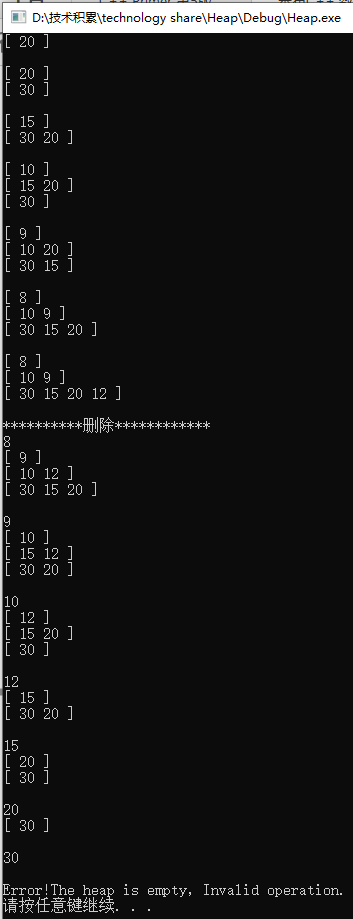上面就是C++实现的大小顶堆。

如果有疑问，欢迎评论区下方留言；本人水平有限 ，如有错误，也欢迎在评论区下方批评指正。若是喜欢本文，就帮忙点赞吧！

展开全文数据结构
• //交换m,i } } 删除堆顶元素中，删除堆顶元素实际上就是交换堆顶和最后一个元素后让长度减 1 1 1。然后从堆顶 i = 1 i=1 i=1开始向下调整： 找到 i i i最大的孩子结点编号 i c ic ic 比较 i i i与 i c ic ic...数据结构 算法
• ## 大顶堆： ## 小顶堆； 1. empty() 如果优先队列为空，则返回真； 2. pop() 删除第一个元素； 3. push() 加入一个元素； 4. size() 返回优先队列中拥有的元素的个数； 5. top() 返回优先队列中有最高...queue
• 按照的特点可以把分为大顶堆和小顶堆大顶堆：每个结点的值都大于或等于其左右孩子结点的值； 小顶堆：每个结点的值都小于或等于其左右孩子结点的值。 （的这种特性非常的有用，常常被当做优先队列使用...排序算法 leetcode 算法
• 构建大顶堆排序实现(java)构建大顶堆排序实现(java)排序介绍：①排序是利用的数据结构设计的一种排序算法，排序是一种选择排序，时间复杂度为O(nlogn)，是不稳定排序；②是具有以下性质的完全...
• 最小（小顶堆）是一种二叉树，树中每个节点都小于他的所有子节点，在最小的构建和维护过程中最重要的是**上浮(swim)和下沉(sink)**操作。 MinHeap.h #include <algorithm> /* 最小类*/ template<...
• ## 大顶堆（c++实现）

千次阅读 2015-07-14 17:00:46
大顶堆的插入：首先初始化插入位置为最后，然后从下往上调整（调整插入元素的位置）。在调整过程中，若当前节点的父亲节点小于插入元素，则将其父亲节点的值赋给当前节点，父亲节点作为当前节点，依此继续；否则...
• 在STL中，默认情况下（不加后面两个参数）是以vector为容器，以 operator为比较方式，所以在只使用第一个参数时，优先队列默认是一个最大，每次输出的堆顶元素是此时中的最大元素。 成员函数 假设type类型为int...
• /*如果存在右子节点且左子节点小于右子节点，将child更新为右子节点*/ } if(lastdata[child]){/*如果待插入值，即数组最后一个节点，小于child的值，就将child上浮（因为大顶堆需要的值在上面）*/ H->data[i]=H->...数据结构 c语言
• 中插入元素有两种情况，一种是为空，那么就让插入值作为根节点即可；另一种是不为空，那么此时就要进行判断当前节点与其父节点的大小关系比较。此时仍有两种情况，一种是当前节点大于父节点，这样正是我们所...
• 如果想堆顶为最小值，则greater替换，并包含头文件#include ，且三个参数全部要写出来 例子： 1.降序输出 #include #include using namespace std; int main(){ priority_queue<int> q; for( int i= 0; i; ...
• #include &lt;iostream&gt; #include &lt;vector&gt; using namespace std;...//大顶堆插入一个数：先将要插入的数存放在的最后（即容器的末尾，下标为index）， // 再与其父节点（下标为(...
• c++算法库中的使用2.1介绍2.2使用案例：3.最大(小)实例应用参考 1.原理 最大最小的定义： 它是一颗完全二叉树,它可以是空 树中结点的值总是不小于(不大于)其孩子结点的值 每一个结点的子树也是一个 当父...
• 一般我们常用的指的是大顶堆是一棵完全二叉树（简单的说，就是前n-1层是满二叉树，最后一层可以没有填充满，全部聚集在左侧的二叉树），树中的每个结点的值都不小（或不大于）其左右孩子结点的值。 其中，...数据结构
• C++ | STL | 大顶堆 | 小顶堆 | std::priority_queue 目录 C++ | STL | 大顶堆 | 小顶堆 | std::priority_queue 1.C++ greater()和less() 1.1.greater()和less()定义 1.2.greater()和less()使用方法 2....
• 是一个基于优先级的无界优先级队列，底层实现是一棵完全二叉树 不允许使用 null 元素 不允许插入不可比较的对象，会导致 ClassCastException。 优先级队列是无界的，但是有一个内部容量，默认容量为11，控制着用于...java
• 其实无论是大顶堆还是小顶堆，会了一个就会另外一个的写法了，这个其实并不难写，我的理解是：所谓插入其实就是往上渗透，所谓删除其实就是往下渗透，他们都是在找最后一个点的合理位置！下面直接上代码了，相关解释...堆栈
• 当父节点的键值总是大于或等于任何一个子节点的键值时为最大(大顶堆)。 当父节点的键值总是小于或等于任何一个子节点的键值时为最小(小顶堆)。根据二叉树的性质，可得： 1. 如果根节点在数组的位置是1,第n个...
• c++堆两种方式 1.优先队列priority_queue 2.make_heap() 下面分别来介绍常用用法： 1. 创建大顶堆： priority_queue <int,vector<int>,less<int> >q; 创建小顶堆： priority_queue <...开发语言 后端
• 在前面的几篇文章中，介绍了线性表的三种数据结构：链表、队列和栈。他们因为各自的特性，都可以方便的支持某一种运算。比如链表相比于数组，其插入和删除...下面给出大顶堆的定义：  一个（二叉）是一棵几乎完全的数据结构
• 是具有以下特性的完全二叉树，每个结点的值都大于或等于其左右孩子结点的值，叫做最大；每个结点的值都小于或等于其左右孩子结点的值，叫做最小。 一、建 vector<int> nums = {9, 6, 2, 4, 7, 0, 1, 8...
• 一、也是一种数据结构，从实际应用意义来说，他是一种最优级别数据永远在第一位的队列，本文皆以最小值为例（小顶堆），即它变相是一种会永远保持最小值先出队的队列。 二、的本质是一颗完全二叉树，树根永远为...acm 算法 数据结构
• 是一种特别的完全二叉树，给定中任意节点P和C，若P是C的母节点，那么P的值会小于等于（或大于等于）C的值”。若母节点的值恒小于等于子节点的值，此称为最小（min heap）；反之，若母节点的值恒大于等于子...队列 数据结构
• ## C++ STL自带的堆

千次阅读 2019-09-08 03:30:52
一、STL自带：priority_queue stack与queue注意事项： 1 stack不允许有遍历行为，stack也不提供迭代器。SGI STL便以deque作为缺省情况下stack底部结构，称之为adapter（配接器） 2 除了deque之外，list也是双向...
• 这里主要练习在不使用 C++ STL 模板 priority_queue 的情况下，纯手工建立优先队列（大小顶堆），然后进行排序做这道题。 在此之前极力建议看下这位博主写的文章 ???? 什么是大小顶堆？ ，再结合我写的实现代码，...leetcode 排序算法 算法...

# c++大顶堆删除堆顶元素c++ 订阅